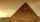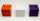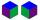# Addition + cube - math problems

#### Number of problems found: 3

• TowerCharles built a tower of cubes with an edge 2 cm long. In the lowest layer there were 6 cubes (in one row) in six rows, in each subsequent layer always 1 cube and one row less. What volume in cm3 did the whole tower have?
• Three cubesThe body was created by gluing three identical cubes. Its volume is 192 cm3. What is its surface in dm2?
• Cube containersTwo containers shaped of cube with edges of 0.7 m and 0.9 m replace a single cube so that it has the same volume as the original two together. What is the length of the edges of the new cube?

We apologize, but in this category are not a lot of examples.
Do you have an exciting math question or word problem that you can't solve? Ask a question or post a math problem, and we can try to solve it.

We will send a solution to your e-mail address. Solved examples are also published here. Please enter the e-mail correctly and check whether you don't have a full mailbox.